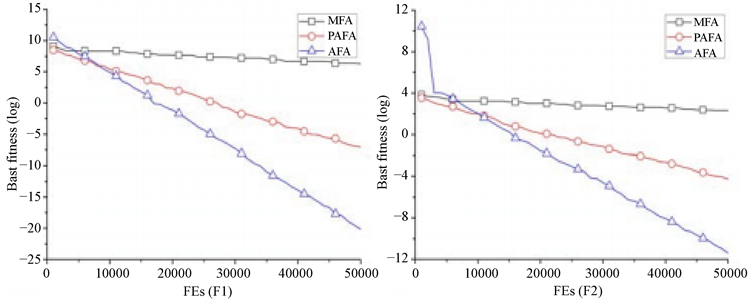# 一种基于自适应搜索策略的改进萤火虫算法An Improved Firefly Algorithm Based on Adaptive Search Strategies

DOI: 10.12677/CSA.2020.109173, PDF, HTML, XML, 下载: 156  浏览: 267  国家科技经费支持

Abstract: Firefly algorithm (FA) is a recently proposed optimisation technique, based on swarm intelligence, which has shown good optimisation performance. However, FA suffers from slow convergence and low accuracy of solutions. To improve this case, this paper presents a new firefly algorithm (AFA) by using three hybrid strategies to obtain a good optimisation performance. First, an adaptive parameter method is used to dynamically changing the step factor. Second, AFA uses a modified search strategy and eliminates the concept of attractiveness. So, HFA does not include two parameters, ab-sorption coefficient and initial attractiveness. Third, a concept of opposition-based learning is used for improving the accuracy of the global best solution. Experiments on some benchmark problems show that AFA is superior to mimetic FA (MFA) and probabilistic attraction-based FA (PAFA).

1. 标准萤火虫算法

$\beta \left({r}_{ij}\right)=\beta {\beta }_{0}{\text{e}}^{-\gamma {r}_{ij}^{2}}$ (1)

${r}_{ij}=‖{X}_{i}-{X}_{j}‖=\sqrt{{\sum }_{d=1}^{D}{\left({x}_{id}-{x}_{jd}\right)}^{2}}$ (2)

${x}_{id}\left(t+1\right)={x}_{id}\left(t\right)+{\beta }_{0}{\text{e}}^{-\gamma {r}_{ij}^{2}}\left({x}_{jd}\left(t\right)-{x}_{id}\left(t\right)\right)+\alpha {\epsilon }_{id}\left(t\right)$ (3)

2. 改进算法的实现

1) 自适应搜索策略

$\alpha \left(t+1\right)={\left(\frac{1}{9000}\right)}^{1/t}\alpha \left(t\right)$ (4)

${x}_{id}\left(t+1\right)={x}_{id}\left(t\right)+{r}_{1d}\left({x}_{jd}\left(t\right)-|{x}_{id}\left(t\right)|\right)-{r}_{2d}\left({x}_{wd}\left(t\right)-|{x}_{id}\left(t\right)|\right)$ (5)

2) 反向学习过程

${X}_{best}^{*}\left(t\right)=\stackrel{¯}{a}+\stackrel{¯}{b}-{X}_{best}\left(t\right)$ (6)

3) 算法实现过程

Begin

Initialise the population;

while the stopping condition is satisfied do

Update the step factor according to equation (4);

for i = 1 to N do

for j = 1 to N do

if f(Xj) < f(Xi) then

Conduct the movement according to equation (5);

Compute the fitness value of Xi;

end if

end for

Conduct the Xbest* according to equation (6);

ifXbest* be better than Xbest

Xbest = Xbest*

end if

end for

end while

End

3. 使用基准函数来测试AFA算法的性能

3.1. 测试函数

$\text{F}1\left(X\right)={\sum }_{i=1}^{D}{x}_{i}^{2},\text{\hspace{0.17em}}\text{\hspace{0.17em}}{x}_{i}\in \left[-100,100\right]$ (7)

$\text{F}2\left(X\right)={\sum }_{i=1}^{D}|{x}_{i}|+{\prod }_{i=1}^{D}{x}_{i},\text{\hspace{0.17em}}\text{\hspace{0.17em}}{x}_{i}\in \left[-10,10\right]$ (8)

$\text{F}3\left(X\right)={\sum }_{i=1}^{D}{\left({\sum }_{j=1}^{i}{x}_{j}\right)}^{2},\text{\hspace{0.17em}}\text{\hspace{0.17em}}{x}_{i}\in \left[-100,100\right]$ (9)

$\text{F}4\left(X\right)={\mathrm{max}}_{i}\left(|{x}_{i}|,1\le i\le D\right),\text{\hspace{0.17em}}\text{\hspace{0.17em}}{x}_{i}\in \left[-100,100\right]$ (10)

$\text{F}5\left(X\right)={\sum }_{i=1}^{D-1}\left(100{\left({x}_{i}-{x}_{i}^{2}\right)}^{2}+{\left({x}_{i}-1\right)}^{2}\right),\text{\hspace{0.17em}}\text{\hspace{0.17em}}{x}_{i}\in \left[-30,30\right]$ (11)

$\text{F}6\left(X\right)={\sum }_{i=1}^{D}i{x}_{i}^{4}+rand\left(0,1\right),\text{\hspace{0.17em}}\text{\hspace{0.17em}}{x}_{i}\in \left[-1.28,1.28\right]$ (12)

$\text{F}7\left(X\right)=418.9829\cdot D-{\sum }_{i=1}^{D-1}\left({x}_{i}\mathrm{sin}\left(\sqrt{|{x}_{i}|}\right)\right),\text{\hspace{0.17em}}\text{\hspace{0.17em}}{x}_{i}\in \left[-500,500\right]$ (13)

3.2. 测试结果分析Table 1. Computational results of each algorithm for D = 10Table 2. Computational results of each algorithm for D = 30(a) (b)

Figure 1. The search processes of AFA, MFA, and PAFA for D = 10, (a) function F1; (b) function F2(a) (b)

Figure 2. The search processes of AFA, MFA, and PAFA for D = 30, (a) function F1; (b) function F2

4. 结束语

  Wang, H., Wang, W.J., Sun, H. and Rahnamayan, S. (2016) Firefly Algorithm with Random Attraction. International Journal of Bio-Inspired Computation, 8, 33-41. https://doi.org/10.1504/IJBIC.2016.074630  Rao, R. (2016) Jaya: A Simple and New Optimization Algorithm for solving Constrained and Unconstrained Optimization Problems. International Journal of Industrial Engineering Computations, 7, 19-34. https://doi.org/10.5267/j.ijiec.2015.8.004  Cai, X.J., Gao, X.Z. and Xue, Y. (2016) Improved Bat Algorithm with Optimal Forage Strategy and Random Disturbance Strategy. International Journal of Bio-Inspired Computation, 8, 205-214. https://doi.org/10.1504/IJBIC.2016.078666  Wang, H., Sun, H., Li, C.H., Rahnamayan, S. and Pan, J.S. (2013) Diversity Enhanced Particle Swarm Optimization with Neighborhood Search. Information Sciences, 223, 119-135. https://doi.org/10.1016/j.ins.2012.10.012  Fister Jr., I., Yang, X.S., Fister, I. and Brest, J. (2012) Memetic Firefly Algorithm for Combinatorial Optimization. Bioinspired Optimization Methods and Their Applications, 103, 1-14.  Yu, G. (2016) An improved Firefly Algorithm Based on Probabilistic Attraction. International Journal of Computing Science and Mathematics, 7, 530-536. https://doi.org/10.1504/IJCSM.2016.081701  Yu, G. and Feng, Y.Y. (2018) Improving Firefly Algorithm Using Hybrid Strategies. International Journal of Computing Science and Mathematics, 9, 163-170. https://doi.org/10.1504/IJCSM.2018.091749  Zhang, M.Q., Wang, H., Cui, Z.H. and Chen, J.J. (2018) Hybrid Multi-Objective Cuckoo Search with Dynamical Local Search. Memetic Computing, 10, 199-208. https://doi.org/10.1007/s12293-017-0237-2  Wang, F., Zhang, H., Li, K., Lin, Z., Yang, J. and Shen, X.-L. (2018) A Hybrid Particle Swarm Optimization Algorithm Using Adaptive Learning Strategy. Information Sciences, 436-437, 162-177. https://doi.org/10.1016/j.ins.2018.01.027  Wang, F., Zhang, H., Li, Y., Zhao, Y. and Rao, Q. (2018) External Archive Matching Strategy for MOEA/D. Soft Computing, 22, 7833-7846. https://doi.org/10.1007/s00500-018-3499-9  Cui, Z., Zhangm J., Wang, Y., et al. (2019) A Pigeon-Inspired Optimization Algorithm for Many-Objective Optimization Problems. Science China Information Sciences, 62, Article No. 70212. https://doi.org/10.1007/s11432-018-9729-5